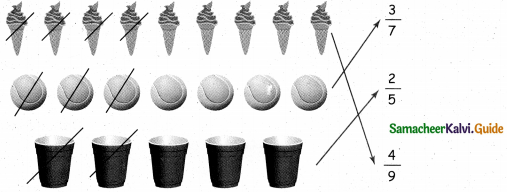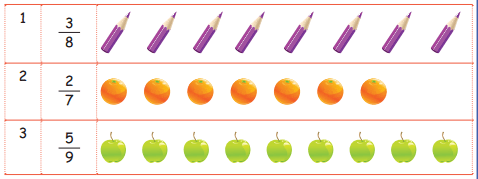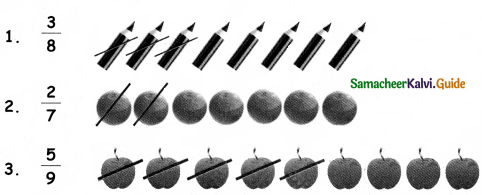Tamilnadu State Board New Syllabus Samacheer Kalvi 4th Maths Guide Pdf Term 2 Chapter 6 Fraction Ex 6.2 Textbook Questions and Answers, Notes.

## Tamilnadu Samacheer Kalvi 4th Maths Solutions Term 2 Chapter 6 Fraction Ex 6.2

Question 1.
Write the fractional part for the pictures that is circled.
(i)$$\frac { 4 }{ 7 }$$(ii)$$\frac { 5 }{ 8 }$$

(iii)$$\frac { 3 }{ 9 }$$Question 2.
Match the following: